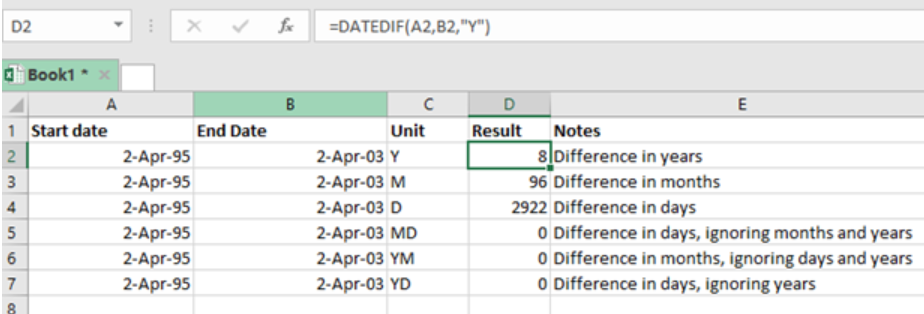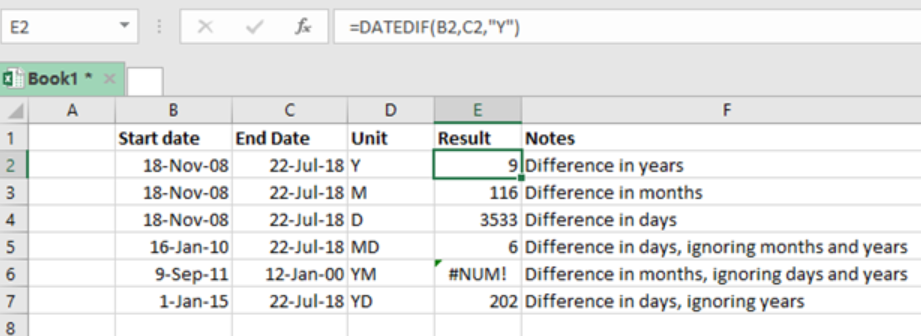Get instant live expert help with Excel or Google Sheets“My Excelchat expert helped me in less than 20 minutes, saving me what would have been 5 hours of work!”

#### Post your problem and you'll get expert help in seconds

Your message must be at least 40 characters
Our professional experts are available now. Your privacy is guaranteed.

# Excel DATEDIF Function

To get the difference between two date values in years, months or even days, we can use the excel DATEDIF function. This is a compatibility function that originates from Lotus 1-2-3. This post provides a clear elaboration on how to use the Excel DATEDIF function to get differences in date values in years, months and days.Figure 1: How find time differences using DATEDIF function

## General syntax of the formula

`=DATEDIF (start_date, end_date, unit)`

Where;

• Start_date– refers to the start date in the excel date serial number format
• End_date– refers to the end date in the excel serial number format
• Unit- time unit in use, (years, months or days)

## Understanding the formula

• This function is only documented in Excel 2000. This means that Excel will not fill out the arguments for you. But if configured correctly, the function works perfectly.
• The function calculates the time in between the start and end dates. This can be in years, months or days.
• The unit argument refers to the time to be returned, and can be supplied as text.
• If the start date is greater than the end date, the DATEDIF function will return a #NUM error.
• To manage the error, especially when the start and end dates are unknown, you can use the IFERROR function.

## ExampleFigure 2: Using DATEDIF function to find time differences

In this example, we want to find the time differences between start time in column B and end time in column C.

• In the result column, which is column E, we shall have the time differences for all the dates indicated.
• In cell E2 we put our formula; =DATEDIF (B2,C2,”Y”)
• We then press Enter to get the time difference for that row.
• We then copy down the formula to get the results for all the other rows, in the result column.

Most of the time, the problem you will need to solve will be more complex than a simple application of a formula or function. If you want to save hours of research and frustration, try our live Excelchat service! Our Excel Experts are available 24/7 to answer any Excel question you may have. We guarantee a connection within 30 seconds and a customized solution within 20 minutes.

### Did this post not answer your question? Get a solution from connecting with the expert.Another blog reader asked this question today on Excelchat:## Subscribe to Excelchat.coAnother blog reader asked this question today on Excelchat: